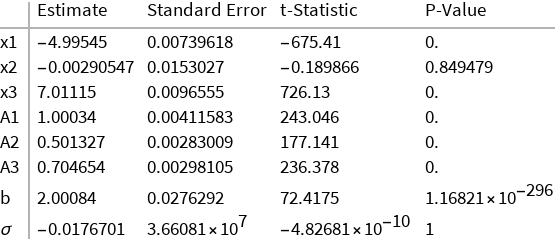#Function Repository Resource:

# NPseudoVoigt

Fast numerical approximation to the PDF of the Voigt distribution with around 1.2% of maximum deviation

Contributed by: Julien Kluge
Quantum Optical Metrology; Joint Lab Integrated Quantum Sensors
Department of Physics
Humboldt-Universität zu Berlin
(julien@physik.hu-berlin.de)
 ResourceFunction["NPseudoVoigt"][x,b,σ] calculates the approximation at postions x of a Voigt distribution with CauchyDistribution parameter b and NormalDistribution parameter σ.

## Details and Options

ResourceFunction["NPseudoVoigt"] is approximately normed to 1 for all parameters b and σ above 0.

## Examples

### Basic Examples

NPseudoVoigt always returns numerical results, even for exact inputs:

 In:=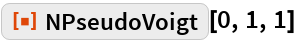Out=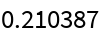NPseudoVoigt compared to the analytic solution:

 In:=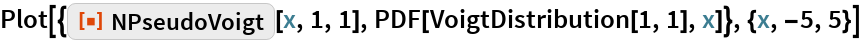Out=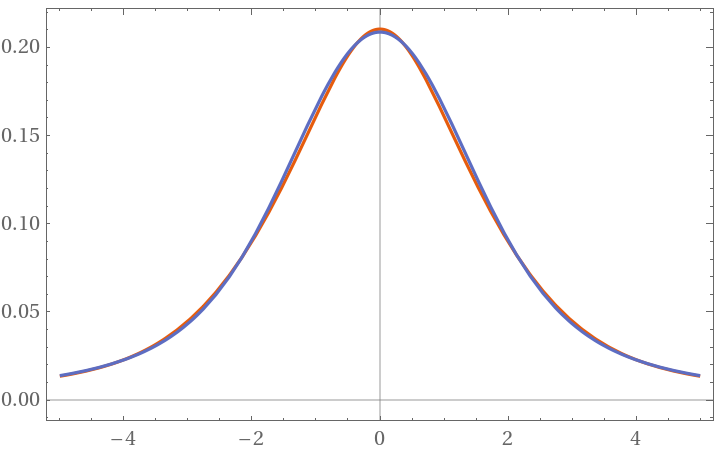### Scope

 In:=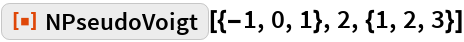Out=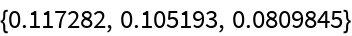NPseudoVoigt is approximately normed to 1 for b and σ greater than 0:

 In:=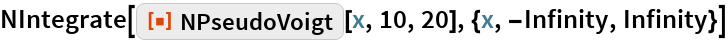Out=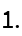NPseudoVoigt's norm has four additional terms that cancel out for non-extreme values of b and σ:

 In:=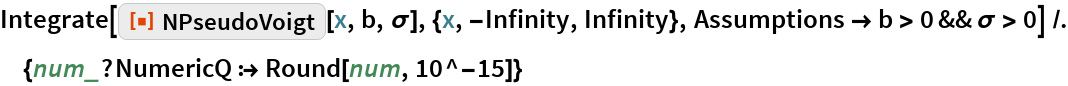Out=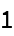NPseudoVoigt's deviation to the analytic solution in the maximum is usually less than ±1% for every parameter combination b and σ:

 In:=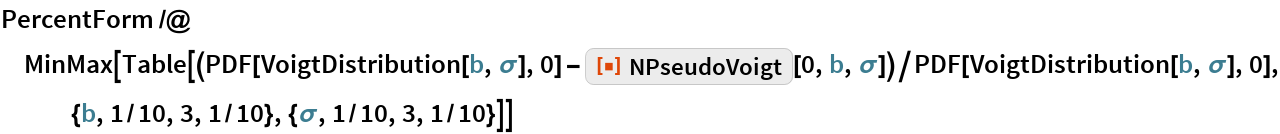Out=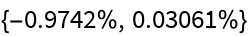NPseudoVoigt's relative deviation increases if b and σ approach one another:

 In:=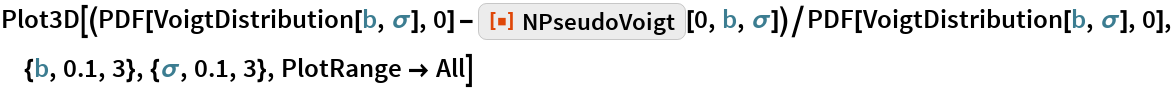Out=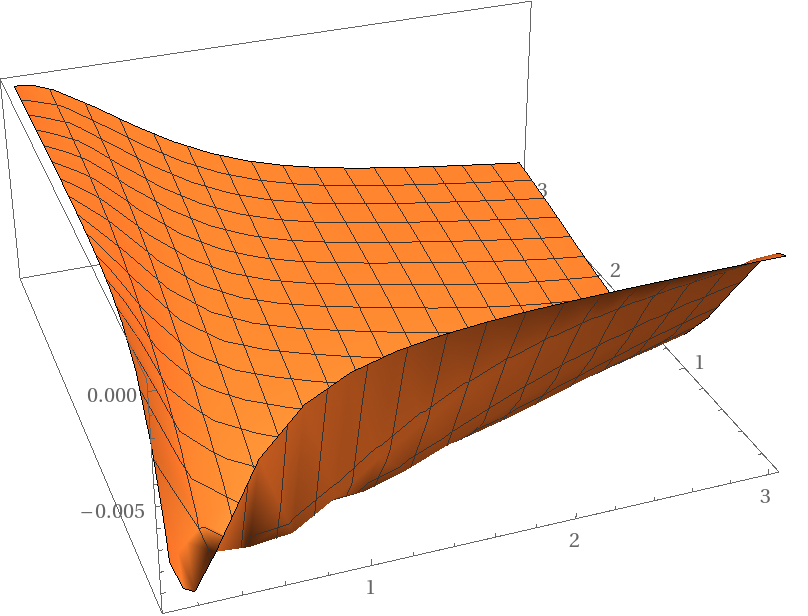NPseudoVoigt is also stable against σ being 0:

 In:=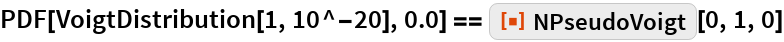Out=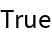### Applications

NPseudoVoigt can be compiled to obtain faster execution times:

 In:=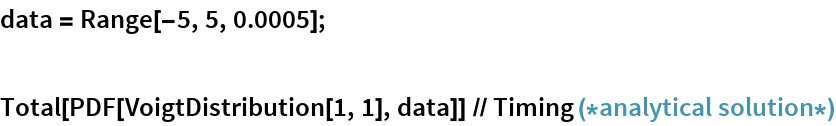Out=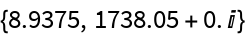In:=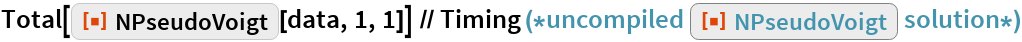Out=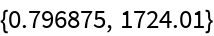In:=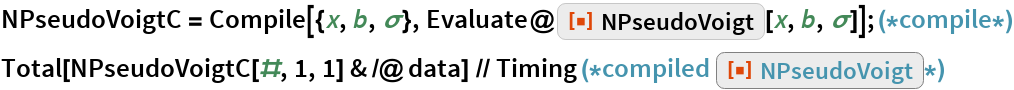Out=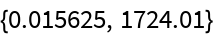### Possible Issues

Negative b parameters can produce imaginary values:

 In:=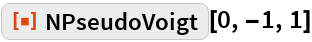Out=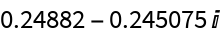Negative σ parameters do not yield symmetric values:

 In:=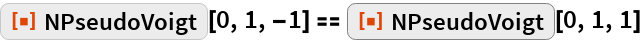Out=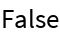### Neat Examples

NPseudoVoigt can be used to approximate an atomic absorption spectrum efficiently:

 In:=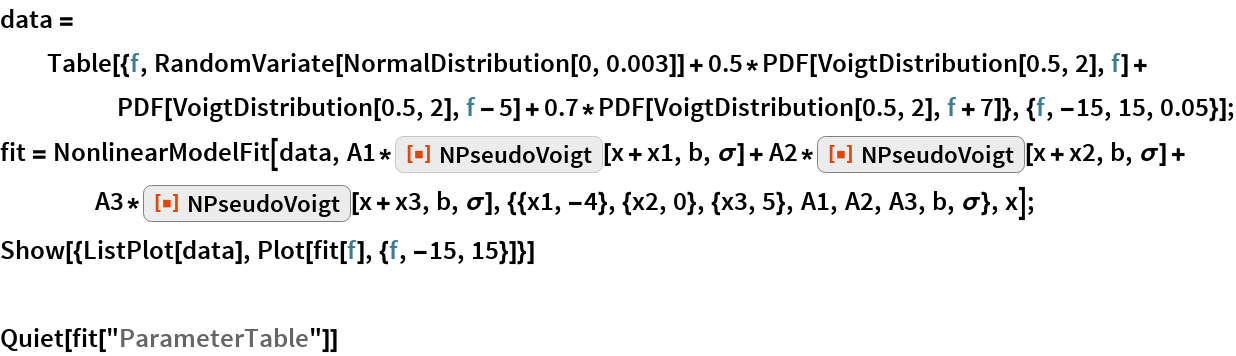Out=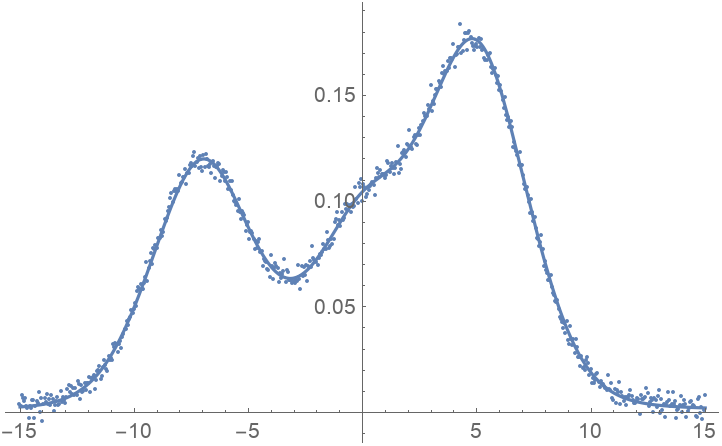Out=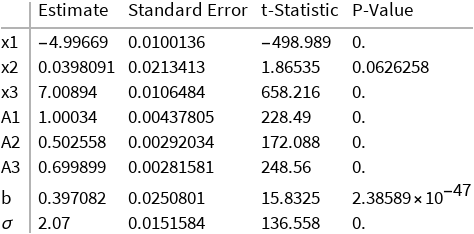NPseudoVoigt can be used to show that σ is negligible or even 0, whereas the VoigtDistribution could throw errors:

 In:=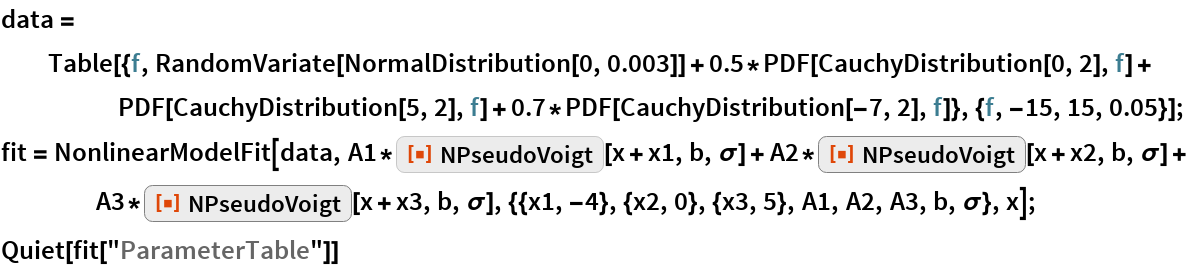Out=